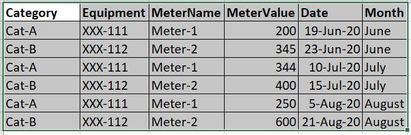cancel
Showing results for
Did you mean:Anonymous
Not applicable

## Volume Calculation

Hi PBI Fox,

I'm struggling to find a solution on how to solve my problem. Can someone help me how to fix it?

I want to get the diff. between each month by category and by meter name. Below is the table.

DATASET.Below, is the report I want to achieve.Your timely response is highly needed.

Regards,

Jhay

1 ACCEPTED SOLUTIONCommunity Support

Hi @Anonymous ,

You may create a measure like DAX below to get diff value per month. While it won't achieve your requirement for the layout of Matrix visual completely, you may try to put [Equipment]and  [MeterName] into Rows box of Matrix, put [Category] into Columns box, put [Month] and [Diff Value per month] into Values box.

``````Diff Value per month =
VAR _PreMonth =
CALCULATE (
SUM ( Table1[MeterValue] ),
FILTER (
ALLEXCEPT ( Table1, [Category], [Equipment], [MeterName] ),
YEAR ( Table1[Date] ) = YEAR ( MAX ( Table1[Date] ) )
&& MONTH ( Table1[Date] )
= MONTH ( MAX ( Table1[Date] ) ) - 1
)
)
VAR _CurMonth =
CALCULATE (
SUM ( Table1[MeterValue] ),
FILTER (
ALLEXCEPT ( Table1, [Category], [Equipment], [MeterName] ),
YEAR ( Table1[Date] ) = YEAR ( MAX ( Table1[Date] ) )
&& MONTH ( Table1[Date] ) = MONTH ( MAX ( Table1[Date] ) )
)
)
RETURN
_CurMonth - _PreMonth
``````

Best Regards,

Amy

Community Support Team _ Amy

If this post helps, then please consider Accept it as the solution to help the other members find it more quickly.

6 REPLIES 6Community Support

Hi @Anonymous ,

You may create a measure like DAX below to get diff value per month. While it won't achieve your requirement for the layout of Matrix visual completely, you may try to put [Equipment]and  [MeterName] into Rows box of Matrix, put [Category] into Columns box, put [Month] and [Diff Value per month] into Values box.

``````Diff Value per month =
VAR _PreMonth =
CALCULATE (
SUM ( Table1[MeterValue] ),
FILTER (
ALLEXCEPT ( Table1, [Category], [Equipment], [MeterName] ),
YEAR ( Table1[Date] ) = YEAR ( MAX ( Table1[Date] ) )
&& MONTH ( Table1[Date] )
= MONTH ( MAX ( Table1[Date] ) ) - 1
)
)
VAR _CurMonth =
CALCULATE (
SUM ( Table1[MeterValue] ),
FILTER (
ALLEXCEPT ( Table1, [Category], [Equipment], [MeterName] ),
YEAR ( Table1[Date] ) = YEAR ( MAX ( Table1[Date] ) )
&& MONTH ( Table1[Date] ) = MONTH ( MAX ( Table1[Date] ) )
)
)
RETURN
_CurMonth - _PreMonth
``````

Best Regards,

Amy

Community Support Team _ Amy

If this post helps, then please consider Accept it as the solution to help the other members find it more quickly.Anonymous
Not applicable

Hi Team,

I tried it but still it didn't work, I really don't know why? 😭 I am just 2 weeks in the learning process of PBI, so please have a forbearance on me 😢. Can anyone guide me on how to do it, I am begging please? Thanks.

Regards,

JhayCommunity Support

Hi @Anonymous ,

It is impossible to achieve the layout completely as you showed in original Post above, so you may try the method which I have suggested, create the measure, then put related fields into Rows , Columns and Values box of Matrix visual.

Best Regards,

Amy

Community Support Team _ Amy

If this post helps, then please consider Accept it as the solution to help the other members find it more quickly.Super User

@Anonymous See my article on Mean Time Between Failure (MTBF) which uses EARLIER: http://community.powerbi.com/t5/Community-Blog/Mean-Time-Between-Failure-MTBF-and-Power-BI/ba-p/339586.
The basic pattern is:
Column =
VAR __Current = [Value]
VAR __PreviousDate = MAXX(FILTER('Table','Table'[Date] < EARLIER('Table'[Date])),[Date])

VAR __Previous = MAXX(FILTER('Table',[Date]=__PreviousDate),[Value])
RETURN
__Current - __Previous

Become an expert!: Enterprise DNA
External Tools: MSHGQM
Latest book!:
Mastering Power BI 2nd EditionDAX is easy, CALCULATE makes DAX hard...Super User

@Anonymous , you can use time intelligence with date table for that

example

``````MTD Sales = CALCULATE(SUM(Sales[Sales Amount]),DATESMTD('Date'[Date]))
last MTD Sales = CALCULATE(SUM(Sales[Sales Amount]),DATESMTD(dateadd('Date'[Date],-1,MONTH)))
last month Sales = CALCULATE(SUM(Sales[Sales Amount]),previousmonth('Date'[Date]))
last MTD (complete) Sales =  CALCULATE(SUM(Sales[Sales Amount]),DATESMTD(ENDOFMONTH(dateadd('Date'[Date],-1,MONTH))))
previous month value =  CALCULATE(sum('table'[total hours value]),previousmonth('Date'[Date]))

diff = [MTD Sales]-[last MTD Sales]
diff % = divide([MTD Sales]-[last MTD Sales],[last MTD Sales])``````

To get the best of the time intelligence function. Make sure you have a date calendar and it has been marked as the date in model view. Also, join it with the date column of your fact/s. Refer :
https://www.archerpoint.com/blog/Posts/creating-date-table-power-bi
https://www.sqlbi.com/articles/creating-a-simple-date-table-in-dax/

See if my webinar on Time Intelligence can help: https://community.powerbi.com/t5/Webinars-and-Video-Gallery/PowerBI-Time-Intelligence-Calendar-WTD-YTD-LYTD-Week-Over-Week/m-p/1051626#M184Anonymous
Not applicable

Hi, I tried it but it didn't work. 😢